# Trying to understand Integral nonlinearity in DAC/ADC

#### asdasd12e12

Joined Nov 24, 2021
20
So here's the definition of INL: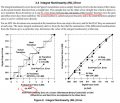it is mentioned (underlined in red) that one of the lines can be a line drawn between the end points of the transfer function but in (a) that line appears to pass in the center of the perfect transfer function and not in the end points, am i missing something?

Same thing happens here: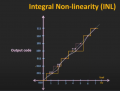in this case the INL is defined as the deviation of the mid-points of the quantization steps between the ideal and real transfer function, where the ideal transfer function is exactly situated in the mid points of the perfect transfer function (not the end points).

In the first image there's also this:

"The name integral nonlinearity derives from the fact that the summation of the differential nonlinearities from the bottom up to a particular step, determines the value of the integral nonlinearity at that step."

How so? If i add the previous 2 errors in the last image i provided here i will get the next error?

Also in these 2 images :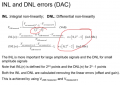and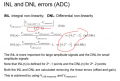Why is the Vlsb measured equal to the difference between two digital voltages divided by 2n -2 in ADC, why not just 2n?

Why is INL only defined for 2n - 1 points for ADC and 2n points for DAC and DNL only defined for 2n -2 for ADC and 2n -1 for DAC?

And last question is related with the picture below: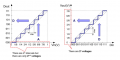Why does the ADC only have 2n -1 voltages?